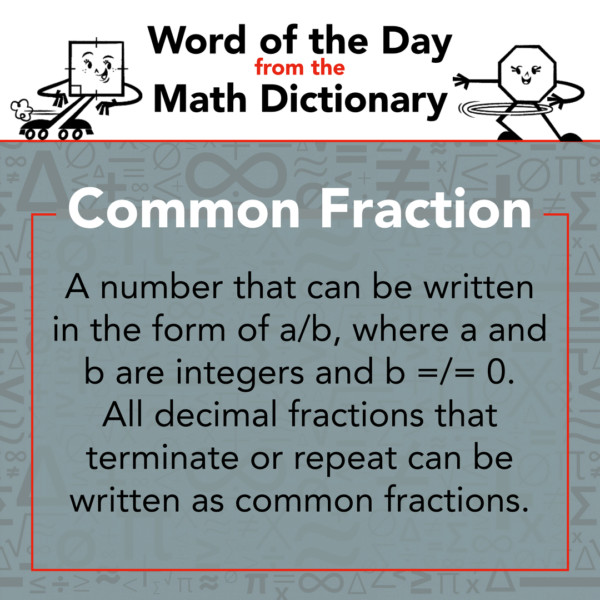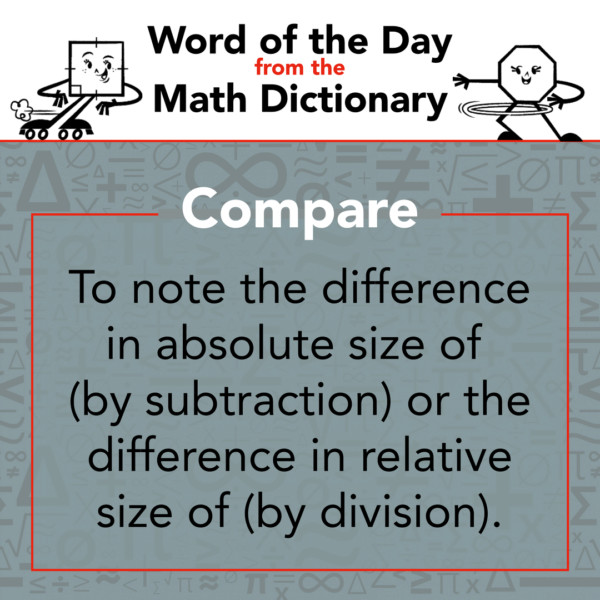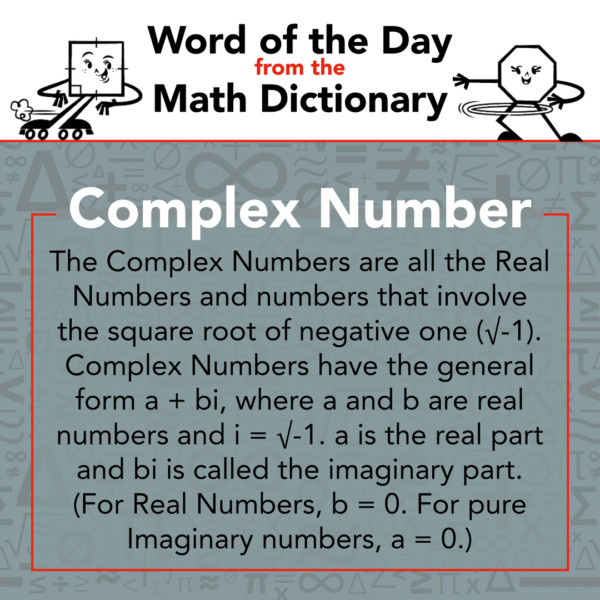877-601-6284
Get Started by Finding a Local Center

# Math Word of the Day - Week of November 8

Nov 8, 2020 | Location 4S Ranch

Math Word of the Day comes from the Math Dictionary written by Mathnasium.

### Common Fraction

Sunday, November 8

A number that can be written in the form of a/b, where a and b are integers and b =/= 0. All decimal fractions that terminate or repeat can be written as common fractions.### Commutative Property

Monday, November 9

The law of mathematics that allows the independence of the ordering of the elements in a problem. Operations (such as addition and multiplication) are commutative because the elements can be operated on in any order without affecting the result.

Examples:

a + b = b + a

ab = ba

Note: Generally, subtraction and division are not commutative. It does matter in what order we subtract and divide (except in special cases).### Compare

Tuesday, November 10

To note the difference in absolute size of (by subtraction) or the difference in relative size of (by division).### Compass

Wednesday, November 11

A device for drawing circles or circular arcs and for making measurements.### Complement

Thursday, November 12

"The rest of it." The quantity necessary to make a complete whole; the remaining part with respect to the whole.### Complex Number

Friday, November 13

The Complex Numbers are all the Real Numbers and numbers that involve the square root of negative one (√-1). Complex Numbers have the general form a + bi, where a and b are real numbers and i = √-1. a is the real part and bi is called the imaginary part. (For Real Numbers, b = 0. For pure Imaginary numbers, a = 0.)### Composite Number

Saturday, November 14

A number with three or more factors.##### Locations near
ME
Capitol Hill DC 28.48 mi
621 Pennsylvania Ave SE
1st-floor unit
Washington, DC 20003